#Function Repository Resource:

# SelectPermutations

Get permutations that satisfy a certain criterion

Contributed by: Sander Huisman
 ResourceFunction["SelectPermutations"][list,crit] generates a list of all possible permutations of the elements in list satisfying crit. ResourceFunction["SelectPermutations"][list,n,crit] gives all permutations containing at most n elements satisfying crit. ResourceFunction["SelectPermutations"][list,{n},crit] gives all permutations containing exactly n elements satisfying crit. ResourceFunction["SelectPermutations"][…,crit,m] gives at most m results.

## Details and Options

There are n! permutations of a list of n distinct elements.
Repeated elements are treated as identical.
The object list need not have head List.
ResourceFunction["SelectPermutations"][list,crit] is effectively equivalent to ResourceFunction["SelectPermutations"][list,{Length[list]},crit].
ResourceFunction["SelectPermutations"][list,{nmin,nmax},crit] gives permutations of between nmin and nmax elements.
ResourceFunction["SelectPermutations"][list,{nmin,nmax,dn},crit] gives permutations nmin, nmin+dn, nmin+2dn … and nmax elements.

## Examples

### Basic Examples

Select from the list {5,6,7,8,9} those permutations that form a prime when concatenating the digits:

 In:=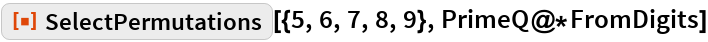Out=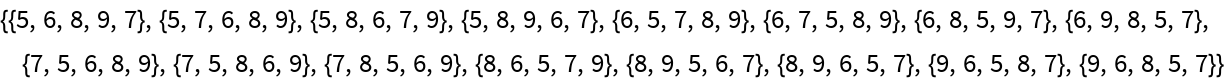Select permutations of length 3:

 In:=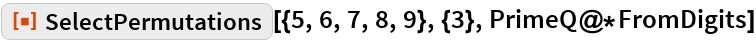Out=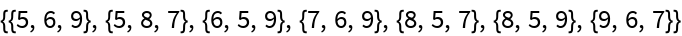Select permutations of length 3–4:

 In:=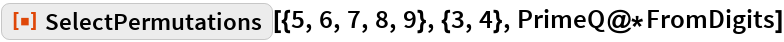Out=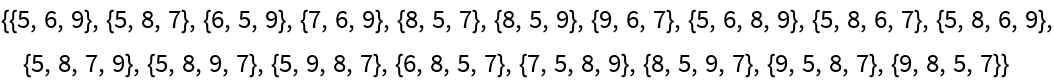Select permutations for which the first two elements and the last elements add up to the same value:

 In:=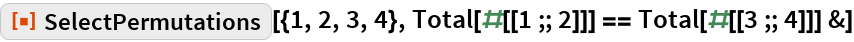Out=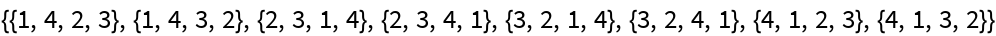### Scope

Select the first 10 permutations of length 4 for which the elements add up to an odd number:

 In:=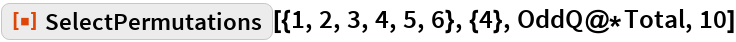Out=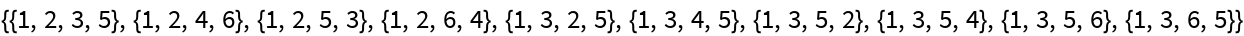### Applications

Select polynomials for which the slope is 1 at x=0:

 In:=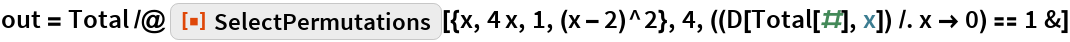Out=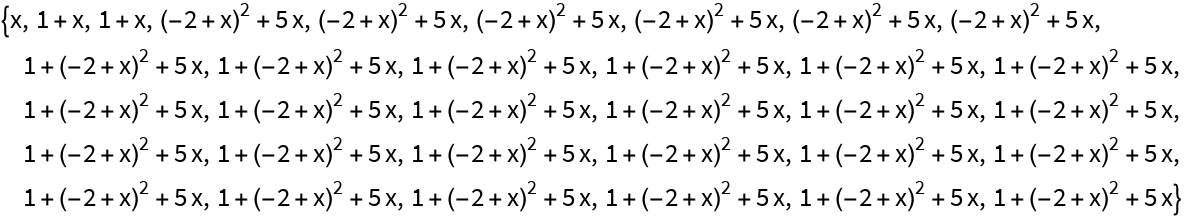Confirm that the slope is indeed unity at x=0:

 In:=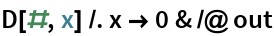Out=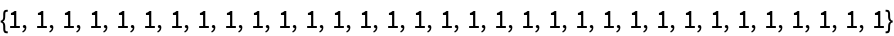### Properties and Relations

Duplicated items are treated the same:

 In:=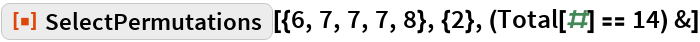Out=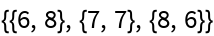The main difference between SelectPermutations[list,crit] and Select[Permutations[list],crit] is the memory usage:

 In:=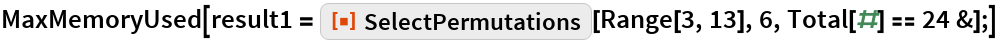Out=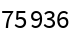Using Select and Permutations uses 500 times more memory:

 In:=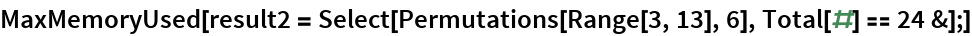Out=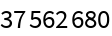Verify that the results are identical:

 In:=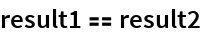Out=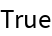Head does not have to be List:

 In:=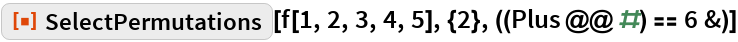Out=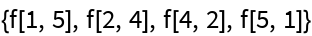### Possible Issues

SelectPermutations might take longer, as it is written in higher-level code as compared to the implementation of Permutations:

 In:=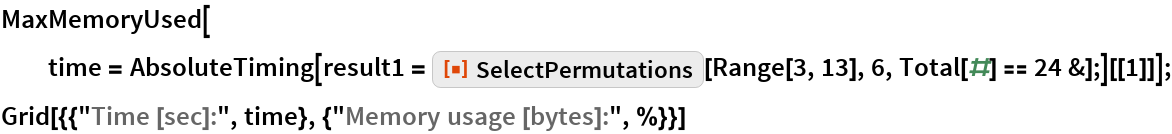Out=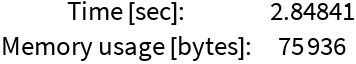Using the built-in functions is faster at the expense of 500× more memory:

 In:=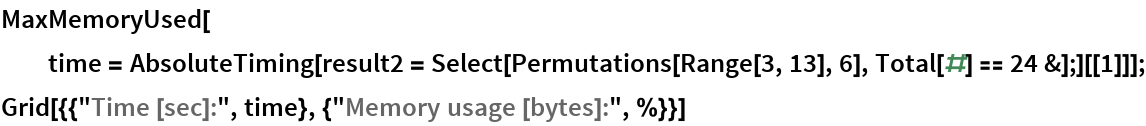Out=